$\require{cancel}$

# Basic Gates

• This is partially from appendix B.
• We are beginning combinational logic or logic which does not contain memory.
• Sequential logic is the opposite, logic that contains a memory.
• I will demo with tkgate because I like it.
• For us, the basic components of logic are
• not
• and
• or
• We will also be using these support devices
• Switches
• LEDs
• tri-state devices
• This appears to be called a driver in Digital.
• Logic is not electronics.
• We are at a weird mixture between the two, but
• Think about vdd as a source of true
• Think about ground as a source of false.
• This is wrong, but it will work.
• Each of the logic component can be expressed three ways
• Boolean Expression
• Truth Table
• Symbol
• You should be able to switch between the three.
• Not
• $f(x) = \overline{x}$
• In logic it might be $f(x) = \neg x$
• $x$ $\overline{x}$
0 1
1 0
•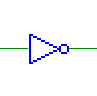• The datasheet for a not chip.
• Generally we think of not as having less than a unit cost.
• and
• $f(a,b) = a \cdot b = ab$
• In logic $f(a,b) = a \land b$
• ab ab
00 0
01 0
10 0
11 1
•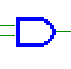• The datasheet for similar chips.
• or
• $f(a,b) = a + b = ab$
• In logic $f(a,b) = a \lor b$
• ab ab
00 0
01 1
10 1
11 1
•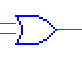• And and or are counted as single units in delay terms.
• There is an exclusive or. (xor)
• nand and nor are inverted versions of and and or.
•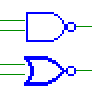• These are just an and or or gate negated.
• It is common to put a dot in place of a inverter or not gate.
• Crossing the wires
• If wires just cross, they do not make a connection.
• If wires have a dot at the intersection, they make a connection.
•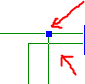• Tristate
• This allows us to control what comes out of a circuit.
• Think putting something on the buss.
• This can be built out of transistors.
•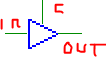• It has
• A data input wire
• A data output wire
• A control line
• If the control line is high, the data from the input is passed to the output.
• If the control line is low, nothing is passed to the output.
• More than two input and/or gates
• For or, if any one is true, the entire thing is true.
• For and, if any one is false, the entire thing is false.
•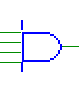• Bundled wires
• Sometimes we wish to run multiple bits through a device.
• We do this with bundled wires.
•• We indicate the number of wires with a slash and a number
• I am sure that I have forgotten something, but we will deal with it as it arises.